## Tuesday, June 30, 2009

### Derivative and Integral of the Heaviside Step Function

The Setup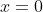the function looks exactly the same.

The Heaviside step function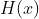, sometimes called the Heaviside theta function, appears in many places in physics, see  for a brief discussion. Simply put, it is a function whose value is zero for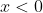and one for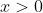. Explicitly,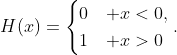(1)
We won't worry about precisely what its value is at zero for now, since it won't effect our discussion, see  for a lengthier discussion. Fig. 1 plots. The key point is that crossing zero flips the function from 0 to 1.

Derivative -- The Dirac Delta Function
Say we wanted to take the derivative of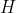. Recall that a derivative is the slope of the curve at at point. One way of formulating this is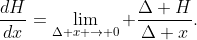(2)
Now, for any pointsor, graphically, the derivative is very clear:is a flat line in those regions, and the slope of a flat line is zero. In terms of (2),does not change, so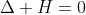and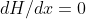. But if we pick two points, equally spaced on opposite sides of, say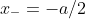and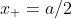, then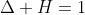and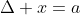. It doesn't matter how small we make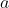,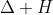stays the same. Thus, the fraction in (2) is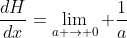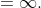(3)
Graphically, again, this is very clear:jumps from 0 to 1 at zero, so it's slope is essentially vertical, i.e. infinite. So basically, we have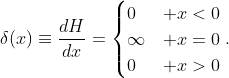(4)
This function is, loosely speaking, a ``Dirac Delta'' function, usually written as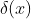, which has seemingly endless uses in physics.

We'll note a few properties of the delta function that we can derive from (4). First, integrating it from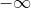to any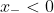: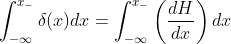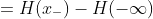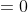(5)
since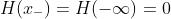. On the other hand, integrating the delta function to any point greater than: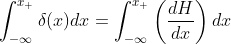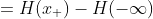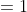(6)
since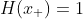.

At this point, I should point out that although the delta function blows up to infinity at, it still has a finite integral. An easy way of seeing how this is possible is shown in Fig. 2(a). If the width of the box is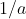and the height is, the area of the box (i.e. its integral) is, no matter how largeis. By lettinggo to infinity we have a box with infinite height, yet, when integrated, has finite area.

Integral -- The Ramp Function
Now that we know about the derivative, it's time to evaluate the integral. I have two methods of doing this. The most straightforward way, which I first saw from Prof. T.H. Boyer, is to integratepiece by piece. The integral of a function is the area under the curve,1 and whenthere is no area, so the integral fromto any point less than zero is zero. On the right side, the integral to a point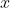is the area of a rectangle of height 1 and length, see Fig. 1(a). So, we have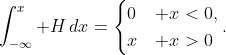(7)
We'll call this function a ``ramp function,''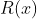. We can actually make use of the definition ofand simplify the notation: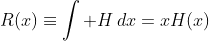(8)
since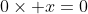and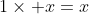. See Fig. 2(b) for a graph -- and the reason for calling this a ``ramp'' function.

But I have another way of doing this which makes use of a trick that's often used by physicists: We can always add zero for free, since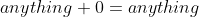. Often we do this by adding and subtracting the same thing,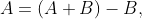(9)
for example. But we can use the delta function (4) to add zero in the form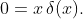(10)
Sinceis zero for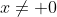, thepart doesn't do anything in those regions and this expression is zero. And, although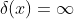at,at, so the expression is still zero.

So we'll add this on to: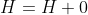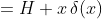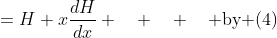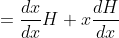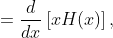(11)
where the last step follows from the ``product rule'' for differentiation. At this point, to take the integral of a full differential is trivial, and we get (8).

References
 M. Springer. Sunday function [online]. February 2009. Available from: http://scienceblogs.com/builtonfacts/2009/02/sunday_function_22.php [cited 30 June 2009].
 E.W. Weisstein. Heaviside step function [online]. Available from: http://mathworld.wolfram.com/HeavisideStepFunction.html [cited 30 June 2009].

1 To be completely precise, it's the (signed) area between the curve and the line.

1.Not to nit pick, but unless I am misunderstanding the entire post (not to mention verious math, physics, and engineering classes I've taken) I believe the line after (6) should read "since H(x+)=1" not "since H(x-)=1"

2.Yup, typo. It's fixed. Thanks for catching it.

3.Thanks a lot for this, very useful for figuring out my assignment.

4.hi,thanks for this,just one question: how did u set x*delta(x)=0 at x=0 as delta(x) in zero would be infinity so x*delta(x)=0*infinity which is NaN?

5.Mehrtash,
It's a bit unrigorous. Basically, the delta function tends towards infinity at x=0, but the area under it is finite, so it's not ``really'' infinity, it's only sort of ``tends to infinity,'' whatever that means. So, 0*(something not really infinite) is 0.

6.This explanation of the integral of the delta function is extremely helpful. In our EE classes they don't give us textbooks with this derivation, and other internet sources just state the result. Thank you for clearing up my misunderstanding.

7.Anonymous - glad I was able to help!

8.Hi, your explanation is very good. But I am looking for the derivative and integration of functions like f(x)H(x) ,e.g., {exp(ax)}H(b-x). If I am not very wrong D{f(x)H(x)}= f'(x)H(x)+f(x){delta(X)} and then by integrating this result I should get back the original function, at this point I am confused how to handle the delta function.
['D' stands for differentiation]

9.Tanay -- that's right. To integrate backwards, first integrate f'(x)H(x) by parts:
Integral[f'(x)H(x)dx] = f(x)H(x) -Integral[f(x)H'(x)dx] = f(x)H(x) - Integral[f(x)delta(x)dx]
This second integral cancels with the Integral[f(x)delta(x)dx], leaving you with f(x)H(x).

10.Could anyone help me understand how you would go about integrating the step function with equation x(t)=u(t+2)-2u(t)+u(t-1) please? I'm trying to draw the ramp function graph from the original step function equation.

11.Eli, thank you very much. I'm in quant this semester and I appreciate this fantastic explanation. I hope I'll find more help from your blog.
Ryan

12.Hey. I have a simple question about your definition over the heaviside function because I saw many definitions that say that H(X) = 1 if x is greater or equal to 0 and not only greater than 0. It changes the following reasonment isn't it ? I can send you a lot of paper where x is greater or equal to 0.

1.